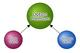# What is 'premium' for options in stock market?

By Research Desk1. Intrinsic Value
2. Time Value

Option premium = Intrinsic value + Time Value

Intrinsic value is the amount by which the strike price of an option is in the money.

Call Option Intrinsic Value = Current Stock Price – Call Strike Price.

If the above value is positive, then the option is ‘In the money’. If it is negative, then the option is ‘out of the money’ and if it zero, it is ‘at the money’

Put Option Intrinsic Value = Put Strike Price - Current Stock Price

If the above value is positive, then the option is ‘Out of the money’. If it is negative, then the option is ‘In the money’ and if it zero, it is ‘at the money’

For more on intrinsic value, refer https://www.sptulsian.com/article/83295/what-is-meant-by-intrinsic-value-

Time value is the difference between premium and intrinsic value. Thus, at-the-money and out-of-money options will have only time value, as their intrinsic value is zero.

For more on time value, refer https://www.sptulsian.com/article/83296/what-is-meant-by-time-value